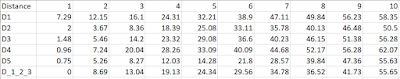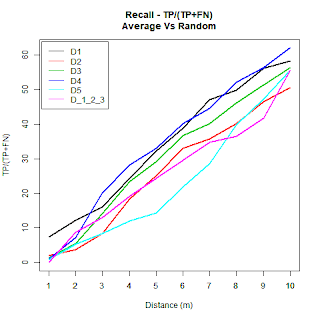## Thursday, 11 January 2018

### R script for generating multi-line graphs from .csv files

Recently I got reviewer's comments on a paper and they asked me to remake my graphs using R. At the beginning I got a bit annoyed that I had to redo them, but the results looks great!! :)  Therefore, I thought about documenting the code within my blog for future reference.

Let assume that you have the following .csv file and you would like to create a multi-line graph (please note that Excel also allows to export spreadsheet into .csv file format).Content of a file table4.csv
The following R script creates a multi-line graphs. The input variables at the beginning can be adjusted accordingly.

### User defined Variables ###
# The title that appears on the top of the graph
mainTitle  = "Recall"
# The tile of the x-axis
xAxisTitle = "Distance (m)"
# The title of the y-axis
yAxisTitle = "Recall"
# The name of the imported .csv file
fileName = "table4.csv"
# The name of the image where the graph will be stored
exportFileName = "table4.png"

# extracts two lists with the labels of the axes
rowNames = row.names(data)
colNames = colnames(data)

png(filename=exportFileName)

# plot graph with the given titles
matplot(t(data), type="l", lty=1, lwd=2, main= mainTitle, xlab=xAxisTitle, ylab=yAxisTitle)

# modify numbering\labels of the axes to agree with the inserted table of the .csv file
axis(1, at=1:length(colNames), lab=colNames)

# Add legend to the table
legend("topleft", inset=0.01, legend=rowNames, col=c(1:6), bg= ("white"), horiz=F, lty=1)
dev.off()


The result is the following graph:The graph created using the R script

#### 1 comment:

1.For changing the size of the plot, you may add parameters in the "png" command as follow:

png(filename=exportFileName, width = 1480, height = 480)